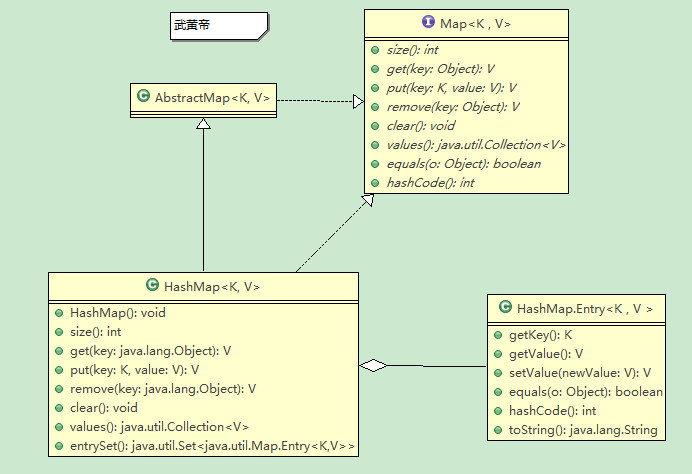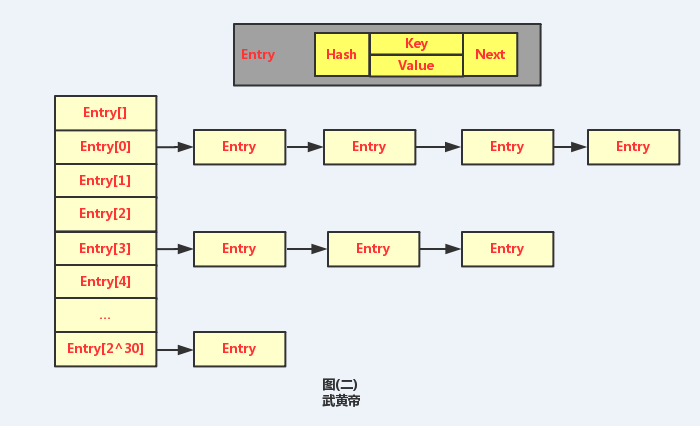## Java-基础-HashMap深度分析 顶转

HashTable是线程安全的，通过synchronized实现的。HashMap取值非常快”等等。这个时候说明他已经很熟练使用HashMap的工具了。

1、HashMap的类图结构

此处的类图是根据JDK1.6版本画出来的。如下图1:2、HashMap存储结构

HashMap的使用那么简单，那么问题来了，它是怎么存储的，他的存储结构是怎样的，很多程序员都不知道，其实当你put和get的时候，稍稍往前一步，你看到就是它的真面目。其实简单的说HashMap的存储结构是由数组和链表共同完成的。如图：3、HashMap基本原理

1、首先判断Key是否为Null，如果为null，直接查找Enrty，如果不是Null，先计算Key的HashCode，然后经过二次Hash。得到Hash值，这里的Hash特征值是一个int值。

2、根据Hash值，要找到对应的数组啊，所以对Entry[]的长度length求余，得到的就是Entry数组的index。

3、找到对应的数组，就是找到了所在的链表，然后按照链表的操作对Value进行插入、删除和查询操作。

4、HashMap概念介绍

size 大小 HashMap的存储大小
threshold 临界值 HashMap大小达到临界值，需要重新分配大小。

loadFactor | 负载因子 | HashMap大小负载因子，默认为75%。|
|modCount | 统一修改 | HashMap被修改或者删除的次数总数。 |
|Entry | 实体 | HashMap存储对象的实际实体，由Key，value，hash，next组成。|

5、HashMap初始化

``````public HashMap(int initialCapacity, float loadFactor) {
// initialCapacity代表初始化HashMap的容量，它的最大容量是MAXIMUM_CAPACITY = 1 << 30。
if (initialCapacity < 0)
throw new IllegalArgumentException("Illegal initial capacity: " +
initialCapacity);
if (initialCapacity > MAXIMUM_CAPACITY)
initialCapacity = MAXIMUM_CAPACITY;

// loadFactor代表它的负载因子，默认是是DEFAULT_LOAD_FACTOR=0.75，用来计算threshold临界值的。
if (loadFactor <= 0 || Float.isNaN(loadFactor))
throw new IllegalArgumentException("Illegal load factor: " +
loadFactor);

// Find a power of 2 >= initialCapacity
int capacity = 1;
while (capacity < initialCapacity)
capacity <<= 1;

this.loadFactor = loadFactor;
threshold = (int)(capacity * loadFactor);
table = new Entry[capacity];
init();
}``````

6、HashMap中的Hash计算和碰撞问题

HashMap的hash计算时先计算hashCode(),然后进行二次hash。代码如下：

``````// 计算二次Hash
int hash = hash(key.hashCode());

// 通过Hash找数组索引
int i = indexFor(hash, table.length);``````

`````` /**
* Returns a hash code for this string. The hash code for a
* <code>String</code> object is computed as
* <blockquote><pre>
* s*31^(n-1) + s*31^(n-2) + ... + s[n-1]
* </pre></blockquote>
* using <code>int</code> arithmetic, where <code>s[i]</code> is the
* <i>i</i>th character of the string, <code>n</code> is the length of
* the string, and <code>^</code> indicates exponentiation.
* (The hash value of the empty string is zero.)
*
* @return  a hash code value for this object.
*/
public int hashCode() {
int h = hash;
if (h == 0 && value.length > 0) {
char val[] = value;

for (int i = 0; i < value.length; i++) {
h = 31 * h + val[i];
}
hash = h;
}
return h;
}``````

The value 31 was chosen because it is an odd prime. If it were even and the multiplication overflowed, information would be lost, as multiplication by 2 is equivalent to shifting. The advantage of using a prime is less clear, but it is traditional. A nice property of 31 is that the multiplication can be replaced by a shift and a subtraction for better performance: 31 * i == (i << 5) - i. Modern VMs do this sort of optimization automatically.

``````static int hash(int h) {
// This function ensures that hashCodes that differ only by
// constant multiples at each bit position have a bounded
// number of collisions (approximately 8 at default load factor).
h ^= (h >>> 20) ^ (h >>> 12);
return h ^ (h >>> 7) ^ (h >>> 4);
}``````

``````/**
* Returns index for hash code h.
*/
static int indexFor(int h, int length) {
return h & (length-1);
}``````

``````  int h=15,length=16;
System.out.println(h & (length-1));
h=15+16;
System.out.println(h & (length-1));
h=15+16+16;
System.out.println(h & (length-1));
h=15+16+16+16;
System.out.println(h & (length-1));``````

``````System.out.println(Integer.parseInt("0001111", 2) & Integer.parseInt("0001111", 2));

System.out.println(Integer.parseInt("0011111", 2) & Integer.parseInt("0001111", 2));

System.out.println(Integer.parseInt("0111111", 2) & Integer.parseInt("0001111", 2));

System.out.println(Integer.parseInt("1111111", 2) & Integer.parseInt("0001111", 2));``````

`````` static int hash(int h) {
// This function ensures that hashCodes that differ only by
// constant multiples at each bit position have a bounded
// number of collisions (approximately 8 at default load factor).
h ^= (h >>> 20) ^ (h >>> 12);
return h ^ (h >>> 7) ^ (h >>> 4);
}``````

``````1. 开放定址法
线性探测再散列，二次探测再散列，伪随机探测再散列）
2. 再哈希法
3. 链地址法
4. 建立一 公共溢出区
``````

7、HashMap的put()解析

`````` /**
* Associates the specified value with the specified key in this map.
* If the map previously contained a mapping for the key, the old
* value is replaced.
*
* @param key key with which the specified value is to be associated
* @param value value to be associated with the specified key
* @return the previous value associated with <tt>key</tt>, or
*         <tt>null</tt> if there was no mapping for <tt>key</tt>.
*         (A <tt>null</tt> return can also indicate that the map
*         previously associated <tt>null</tt> with <tt>key</tt>.)
*/
public V put(K key, V value) {
if (key == null)
return putForNullKey(value);
int hash = hash(key.hashCode());
int i = indexFor(hash, table.length);
for (Entry<K,V> e = table[i]; e != null; e = e.next) {
Object k;
if (e.hash == hash && ((k = e.key) == key || key.equals(k))) {
V oldValue = e.value;
e.value = value;
e.recordAccess(this);
return oldValue;
}
}

modCount++;
addEntry(hash, key, value, i);
return null;
}``````

(1) 首先判断key是否为null，如果是null，就单独调用putForNullKey(value)处理。代码如下：

`````` /**
* Offloaded version of put for null keys
*/
private V putForNullKey(V value) {
for (Entry<K,V> e = table; e != null; e = e.next) {
if (e.key == null) {
V oldValue = e.value;
e.value = value;
e.recordAccess(this);
return oldValue;
}
}
modCount++;
addEntry(0, null, value, 0);
return null;
}``````

(2) 计算key的hashcode，再用计算的结果二次hash，通过indexFor(hash, table.length);找到Entry数组的索引i。

(3) 然后遍历以table[i]为头节点的链表，如果发现有节点的hash，key都相同的节点时，就替换为新的value，然后返回旧的value。

(4) modCount是干嘛的啊? 让我来为你解答。众所周知，HashMap不是线程安全的，但在某些容错能力较好的应用中，如果你不想仅仅因为1%的可能性而去承受hashTable的同步开销，HashMap使用了Fail-Fast机制来处理这个问题，你会发现modCount在源码中是这样声明的。

volatile关键字声明了modCount，代表了多线程环境下访问modCount，根据JVM规范，只要modCount改变了，其他线程将读到最新的值。其实在Hashmap中modCount只是在迭代的时候起到关键作用。

``````private abstract class HashIterator<E> implements Iterator<E> {
Entry<K,V> next;    // next entry to return
int expectedModCount;    // For fast-fail
int index;        // current slot
Entry<K,V> current;    // current entry

HashIterator() {
expectedModCount = modCount;
if (size > 0) { // advance to first entry
Entry[] t = table;
while (index < t.length && (next = t[index++]) == null)
;
}
}

public final boolean hasNext() {
return next != null;
}

final Entry<K,V> nextEntry() {
// 这里就是关键
if (modCount != expectedModCount)
throw new ConcurrentModificationException();
Entry<K,V> e = next;
if (e == null)
throw new NoSuchElementException();

if ((next = e.next) == null) {
Entry[] t = table;
while (index < t.length && (next = t[index++]) == null)
;
}
current = e;
return e;
}

public void remove() {
if (current == null)
throw new IllegalStateException();
if (modCount != expectedModCount)
throw new ConcurrentModificationException();
Object k = current.key;
current = null;
HashMap.this.removeEntryForKey(k);
expectedModCount = modCount;
}

}``````

(5) 如果没有找到key的hash相同的节点，就增加新的节点addEntry(),代码如下：

``````void addEntry(int hash, K key, V value, int bucketIndex) {
Entry<K,V> e = table[bucketIndex];
table[bucketIndex] = new Entry<K,V>(hash, key, value, e);
if (size++ >= threshold)
resize(2 * table.length);
}``````

(6) 如果HashMap大小超过临界值，就要重新设置大小，扩容，见第9节内容。

8、HashMap的get()解析

`````` public V get(Object key) {
if (key == null)
return getForNullKey();
int hash = hash(key.hashCode());
for (Entry<K,V> e = table[indexFor(hash, table.length)];
e != null;
e = e.next) {
Object k;
if (e.hash == hash && ((k = e.key) == key || key.equals(k)))
return e.value;
}
return null;
}``````

9、HashMap的size()解析

HashMap的大小很简单，不是实时计算的，而是每次新增加Entry的时候，size就递增。删除的时候就递减。空间换时间的做法。因为它不是线程安全的。完全可以这么做。效力高。

9、HashMap的reSize()解析

``````void resize(int newCapacity) {
Entry[] oldTable = table;
int oldCapacity = oldTable.length;
if (oldCapacity == MAXIMUM_CAPACITY) {
threshold = Integer.MAX_VALUE;
return;
}

Entry[] newTable = new Entry[newCapacity];
transfer(newTable);
table = newTable;
threshold = (int)(newCapacity * loadFactor);
}``````

``````void transfer(Entry[] newTable) {
Entry[] src = table;
int newCapacity = newTable.length;
for (int j = 0; j < src.length; j++) {
Entry<K,V> e = src[j];
if (e != null) {
src[j] = null;
do {
Entry<K,V> next = e.next;
int i = indexFor(e.hash, newCapacity);
e.next = newTable[i];
newTable[i] = e;
e = next;
} while (e != null);
}
}
}``````### nickles

Java程序员进化为架构师需要掌握的知识

Java程序员进化为架构师掌握的知识： 一：Java知识 1、进制转换 2、Java基本数据类型 面向对象相关知识 3、类、接口、抽象类 this关键字、static关键字、final关键字 方法的参数传递机制 Ja...

andogo
2014/05/16
1K
2

JavaKotlinAndroidLearn 这是一份关于 Java 、Kotlin 、Android 的学习笔记，既包含对基础知识点的介绍，也包含对一些重要知识点的源码解析，笔记的大纲如下所示： Java 重拾Java（0）-基础知...

2018/08/08
0
0

2016/03/10
568
3
Java架构师六大互联网公司面试经历总结

Java架构师面试经历 Java架构师面试经历从58同城——华为 ——招商银行网络中心——金蝶互联网公司GR——苏宁易购 ——蚂蚁金服，看完鬼知道我经历了什么，但是每一次都是成长。本人从2013年...

java知识分子
04/09
0
0

05/15
0
0

2
0

7
0

go4it

3
0

1、Docker - The TLDR(Too Long,Don't Read,Linxu 终端工具 ) Docker是在Linux和Windows上运行的软件。它创建、管理和编排容器。该软件以开源方式开发，在Github上作为Moby开源项目的一部分。...

3
0
Python猫荐书系列之七：Python入门书籍有哪些？

5
0